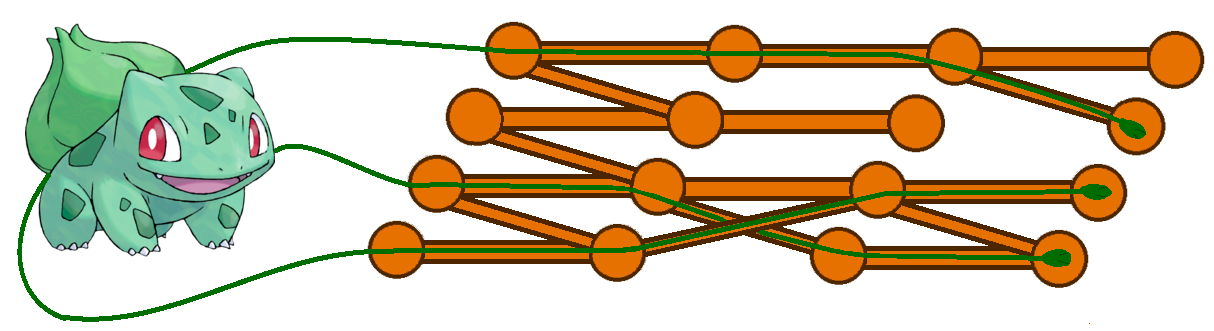시간 제한메모리 제한제출정답맞힌 사람정답 비율
6 초 512 MB555100.000%

## 문제

Bulbasaur is standing next to the field full of holes in the ground. There are n · k holes partitioned into n groups, each consisting of k holes. The groups are numbered with integers from 1 to n and the holes in each group are numbered from 1 to k. There are also some one-directional tunnels between the holes. They can only connect the holes in the i-th group with the holes in the (i + 1)-th group for any i from the range [1, n − 1].

Bulbasaur wants to penetrate the holes and tunnels with its vines. Its vine can enter any hole, go through the tunnels and then finish in some other hole (without going out from the ground). Each taken tunnel must lead from the lower-indexed group to the higher-indexed group. As the holes and tunnels are rather tight, only one vine can be in any hole or any tunnel at the same time.

Let’s denote by f(i, j) the maximum number of vines which Bulbasaur can simultaneously put in some of the holes in the i-th group and which can reach the holes in the j-th group.

$\sum_{i=1}^{n}{\sum_{j=i+1}^{n}{f(i,j)}}$

## 입력

The first line contains two integers n and k (2 ≤ n ≤ 40 000, 1 ≤ k ≤ 9) — the number of groups and the number of holes in each group.

Each of next n − 1 blocks describes the connections between the neighboring groups.

Each block consists of k lines, and each line contains k characters. Blocks are separated with single empty lines.

If there is a tunnel between the p-th hole in the i-th group and the q-th hole in the (i + 1)-th group, then the q-th character in the p-th line of the i-th block is ‘1’. Otherwise, this character is ‘0’.

## 출력

Output the value of the sum described in the task statement.

## 예제 입력 1

4 4
1000
1100
0110
0011

0100
1100
0010
0001

1000
1100
0000
0011


## 예제 출력 1

21


## 예제 입력 2

5 3
000
100
010

000
100
010

010
101
010

010
101
010


## 예제 출력 2

17


## 힌트

Here’s the illustration of the first sample test and a sample way to put 3 vines all the way from the first group to the last group:The holes in each group are numbered bottom-up. It’s impossible to use 4 vines, so f(1, 4) equals 3.

In the second sample test, f(3, 4) = f(4, 5) = f(3, 5) = 2 as Bulbasaur cannot have more than one vine in any hole or tunnel.